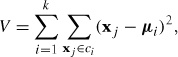# Chapter 6. Clustering Images

This chapter introduces several clustering methods and shows how to use them for clustering images for finding groups of similar images. Clustering can be used for recognition, for dividing data sets of images, and for organization and navigation. We also look at using clustering for visualizing similarity between images.

# 6.1 K-Means Clustering

K-means is a very simple clustering algorithm that tries to partition the input data in k clusters. K-means works by iteratively refining an initial estimate of class centroids as follows:

1. Initialize centroids μi, i = 1 . . . k, randomly or with some guess.

2. Assign each data point to the class ci of its nearest centroid.

3. Update the centroids as the average of all data points assigned to that class.

4. Repeat 2 and 3 until convergence.

K-means tries to minimize the total within-class variancewhere xj are the data vectors. The algorithm above is a heuristic refinement algorithm that works fine for most cases, but it does not guarantee that the best solution is found. To avoid the effects of choosing a bad centroid initialization, the algorithm is often run several times with different initialization centroids. Then the solution with lowest variance V is selected.

The main drawback of this algorithm is that the number of clusters needs to be decided beforehand, and an inappropriate choice will give poor clustering results. The ...

Get Programming Computer Vision with Python now with the O’Reilly learning platform.

O’Reilly members experience live online training, plus books, videos, and digital content from nearly 200 publishers.